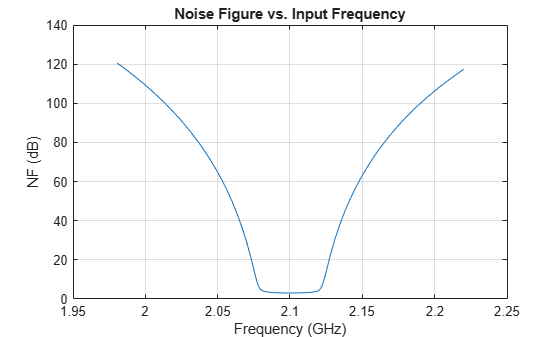# Frequency Sweep in RF Budget Analysis

This example shows how to sweep through frequency-dependent properties of the elements in an RF Budget Analysis.

Use the `nport` and `amplifier` objects to specify the 2-port RF elements in the design and build an RF budget element by cascading the elements together into an RF system with `rfbudget`.

### Building Elements of RF Budget Cascade

Build and parameterize each of the 2-port RF elements.

```f1 = nport('RFBudget_RF.s2p','RFBandpassFilter'); a1 = amplifier('Name','RFAmplifier', ... 'Gain',11.53, ... 'NF',1.53, ... 'OIP3',35);```

Use` rfbudget `object to cascade the elements with input frequency 2.1 GHz, input power -30 dBm, and input bandwidth 45 MHz. This example cascades a filter and an amplifier.

```b = rfbudget('Elements',[f1 a1], ... 'InputFrequency',2.1e9, ... 'AvailableInputPower',-30, ... 'SignalBandwidth',45e6);```

Read frequency-dependent Noise Figure (NF) values of the amplifier from the data-sheet. A similar approach can be followed if the Output third-order intercept (OIP3) or Gain is frequency-dependent.

```% Inputs from the data-sheet freq_datasheet = [1.98;1.99;2.0;2.01;2.02;2.03;2.04;2.05;2.06;2.07;2.08;.... 2.09;2.10].*1e9; NF_datasheet = [1.0000;1.0442;1.0883;1.1325;1.1767;1.2208;1.2650;1.3092;... 1.3533;1.3975;1.4417;1.4858;1.5300]; % Interpolate the amplifier NF data based on existing filter frequencies Freq = f1.NetworkData.Frequencies; RFAmplifier_NF = interp1(freq_datasheet,NF_datasheet,Freq);```

### Plot RF Budget Results Versus Input Frequency

Loop over the desired frequencies, by setting NF of the RF Amplifier element in the `rfbudget` object.

```TotalNF = zeros(size(Freq)); for i = 1:numel(Freq) b.InputFrequency = Freq(i); % Adjust frequency-dependent NF of the RF Amplifier elems(2).NF = RFAmplifier_NF(i); % Compute NF of the cascade TotalNF(i) = b.NF(end); end plot(Freq/1e9,TotalNF) grid on; xlabel('Frequency (GHz)') ylabel('NF (dB)') title('Noise Figure vs. Input Frequency')```